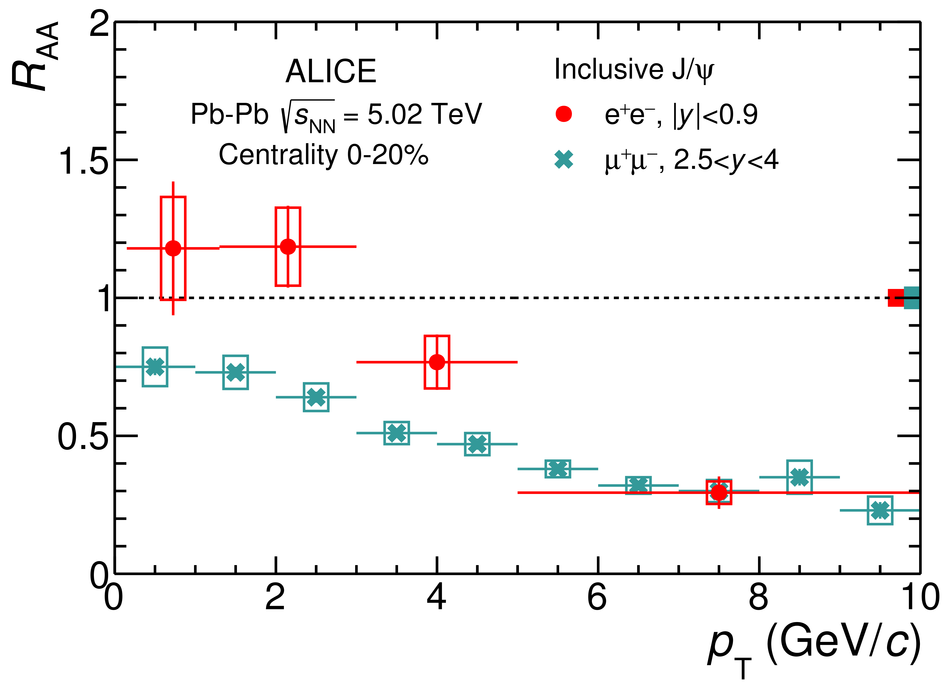# Figure 8

 Left: Inclusive $\jpsi$ $\Raa$ in the $20$\% most central $\PbPb$ collisions at $\snn = 5.02$ TeV as a function of $\pt$, at midrapidity and at forward rapidity . Right: Rapidity dependence of the inclusive $\jpsi$ $\Raa$ in the centrality interval $0$--$90$\%. The error bars represent statistical uncertainties, while the boxes around the data points represent systematic uncertainties. The boxes around unity represent global uncertainties on the $\pp$ reference due to normalization and $\langle\TAA\rangle$. In the right panel, the correlated uncertainty of the point at midrapidity is included in the box around the data point.